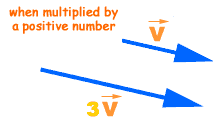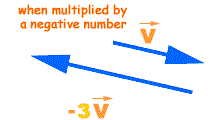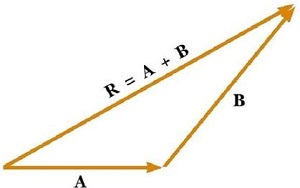# Motion in A Plane - Online Test

Q1. The basic difference between a scalar and vector is one of
Explaination / Solution:

Scalar quantity gives you an idea about how much of an object there is, but vector quantity gives you an indication of how much of an object there is and that also in which direction. So, the main difference between these two quantities is associated with the direction, i.e. scalars do not have direction but vectors do.

Q2. Vectors can be added by
Explaination / Solution:

The sum of two or more vectors is called the resultant. The resultant of two vectors can be found using either the triangle method or the parallelogram method.

Q3. Magnitude of displacement of a particle is
Explaination / Solution:

The maximum possible value for displacement is the distance travelled, so it cannot be of a greater value than distance (path length). The magnitude of the displacement is always less than or equal to the distance traveled. If two displacements in the same direction are added, then the magnitude of their sum will be equal to the distance traveled.

Q4. Two vectors are equal if
Explaination / Solution:

Equal vectors are vectors that have the same magnitude and the same direction. Equal vectors may start at different positions.

Q5.

Multiplying a vector    by a positive real number

Explaination / Solution:

When a vector is multiplied by a positive number (for example 2, 3 ,5, 60 unit etc.) or a scalar only its magnitude is changed but its direction remains the same as that of the original vector.Q6.

Multiplying a vector   by a negative real number

Explaination / Solution:

If a vector is multiplied by a negative number (for example -2, -3 ,-5, -60 unit etc.) or a scalar not only its magnitude is changed but its direction also reversed.Q7. To find the sum of vectors A and B, we place vector B
Explaination / Solution:

Triangle law of vector addition states that when two vectors are represented by two sides of a triangle in magnitude and direction taken in same order then third side of that triangle represents in magnitude and direction the resultant of the vectors.Taken in same order mean tail of Vector B must coincide with head of vector A as shown in image.

Explaination / Solution:

Vector addition is commutative, just like addition of real numbers. If you start from a point say P,  you end up at the same spot no matter which displacement (a or b) you take first. The head-to-tail rule yields vector c for both a + b and b + a.

Q9. The addition of vectors and the multiplication of a vector by a scalar together gives rise to
Explaination / Solution:

If a vector is multiplied by a scalar as in $p\stackrel{}{A}$ ,  then the magnitude of the resulting vector is equal to the product of p and the magnitude of ,   and its direction is the same as $\stackrel{}{A}$ if p is positive and opposite to $\stackrel{}{A}$ if p is negative.

Distributive law for scalar multiplication:

Q10. Null vector or a zero vector has a magnitude
Explaination / Solution:

A null vector is a vector having magnitude equal to zero.It is represented by .

A null vector has no direction or it may have any direction.

Generally a null vector is either equal to resultant of two equal vectors acting in opposite directions or multiple vectors in different directions.